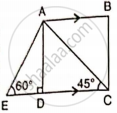# In the Given Figure, Ab and Ec Are Parallel to Each Other. Sides Ad and Bc Are 2 Cm Each and Are Perpendicular to Ab.Given that ∠Aed = 60o and ∠Acd = 45o. Calculate: Ac - Mathematics

Sum

In the given figure, AB and EC are parallel to each other. Sides AD and BC are 2 cm each and are perpendicular to AB.Given that ∠AED = 60° and ∠ACD = 45°. Calculate: AC

#### Solution

Again

sin 45° = "AD"/"AC"

(1)/sqrt(2) = (2)/"AC"

AC = 2sqrt(2).

Concept: Solution of Right Triangles
Is there an error in this question or solution?

#### APPEARS IN

Selina Concise Mathematics Class 9 ICSE
Chapter 24 Solution of Right Triangles [Simple 2-D Problems Involving One Right-angled Triangle]
Exercise 24 | Q 11.2 | Page 304